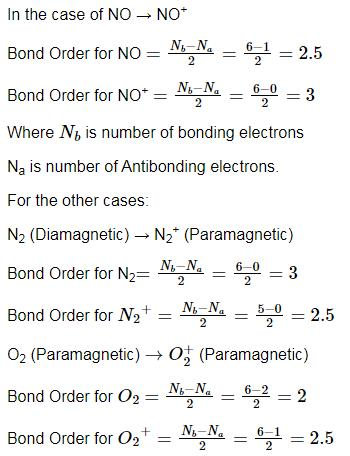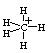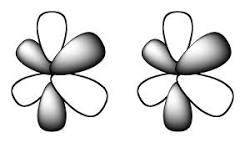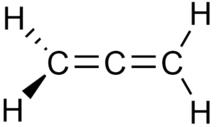Courses

# Test: Chemical Bonding- 1

## 20 Questions MCQ Test Inorganic Chemistry | Test: Chemical Bonding- 1

Description
This mock test of Test: Chemical Bonding- 1 for Chemistry helps you for every Chemistry entrance exam. This contains 20 Multiple Choice Questions for Chemistry Test: Chemical Bonding- 1 (mcq) to study with solutions a complete question bank. The solved questions answers in this Test: Chemical Bonding- 1 quiz give you a good mix of easy questions and tough questions. Chemistry students definitely take this Test: Chemical Bonding- 1 exercise for a better result in the exam. You can find other Test: Chemical Bonding- 1 extra questions, long questions & short questions for Chemistry on EduRev as well by searching above.
QUESTION: 1

### If a bond is made up of a large number of organic compound, then the bond is termed as ______.

Solution:

A covalent bond is a chemical bond that involves the sharing of electron pairs between atoms. Organic Compounds are made up through sharing electron pairs.

QUESTION: 2

### Which compound species has all the carbons, with same kind of hybridization?

Solution:

Ortho, Meta & Para Benzene are all the same. i.e benzene only so all the carbons are having the same kind of hybridization i.e spso, Option D is correct.

QUESTION: 3

### Which among the following formation is not an example of Covalent bond?

Solution:

LiF (Lithium Fluoride) is an example of Ionic bond, as the formation takes place by transfer of electrons and not by sharing.

QUESTION: 4

Which type of bond will be formed by overlapping of dxz and dxz orbitals if the molecular axis is x-axis?

Solution:

Lateral overlap along the X-axis between two dzx orbitals leads to the formation of a Pi-bond.

QUESTION: 5

Examples of refractory materials include:

Solution:

Magnesium Oxide(MgO) is used to make linings for some furnaces. It is known as a refractory material - which just means that it is resistant to heat and retains its strength at very high temperatures.

QUESTION: 6

In which of the following processes, the bond order has increased and paramagnetic character has changed to diamagnetic?

Solution:Diamagnetism is a quantum mechanical effect that occurs in all materials; when it is the only contribution to the magnetism, the material is called diamagnetic.

Paramagnetism is a form of magnetism whereby some materials are weakly attracted by an externally applied magnetic field and form internal induced magnetic fields in the direction of the applied magnetic field.

So, only in the conversion of NO → NO+, the bond order has increased from 2.5 to 3 and the paramagnetic character has changed to diamagnetic.

QUESTION: 7

Which of the following contains a covalent bond?

Solution:
• As we know that ionic bond is formed between an electropositive and an electronegative atom and a covalent bond is formed between two electronegative or electropositive elements.
• In all the above options NO3 the bonds are ionic in nature but NO3 is covalent in nature as bond is formed between two electronegative atoms i.e. N and O.
QUESTION: 8

The hybridization state on BiH3 is:

Solution:

Ammonia adopts sp3 hybridization (HNH bond angle 108°) whereas the other members of the XH3 series PH3, AsH3, SbH3, and BiH3 instead prefer octahedral bond angles of 90-93°.

QUESTION: 9

The hybridization state in triangular bipyramidal CH5+ is:

Solution:
• Carbonium ion methonium,  CH5+ is produced by the addition of  H+ to CH4. In CH5+ three hydrogen atoms are in a plane and one above and one below the plane.• So its sp3d and the shape is trigonal bipyramid.
• But this compound is considered a CH3+ carbenium ion with a molecule of hydrogen interacting with the empty orbital in a
3-center-2-electron bond.
• As the two hydrogen atoms in H2 are in continuously exchange positions with the three hydrogen atoms in the CH3+ methonium CH5+ is considered as a fluxional molecule. Due to the existence of CH3+, this is sp2​ hybridised.
QUESTION: 10

Which among the following chemical bond were described by Kossel and Lewis?

Solution:

Both Ionic and Covalent bond arise from the tendency of atoms to attain stable configuration of electrons.

QUESTION: 11

The direction of dipole moment is correct in:

Solution:

In pyrolle there is greater resonance effect n due to more electronegativity in furan more inductive effect pronounced so the direction of dipole moment in furan is towards oxygen.

QUESTION: 12

If six lobes of one orbital and six lobes of another orbital are overlapped then the resultant bond is:

Solution:In chemistry, phi bonds (φ bonds) are covalent chemical bonds, where six lobes of one involved atomic orbital overlap six lobes of the other involved atomic orbital.

QUESTION: 13

The percentage of p-character in the orbitals forming P−P bonds in P4 is

Solution:

The percentage of p-character in forming P-P bonds in P4 molecule is: 0. In forming
Pmolecule, P atom uses sp3 hybrid orbitals. Thus, the p-character in hybrid orbitals is 75%.

QUESTION: 14

Which has maximum s% in orbital having lp among?

Solution:

Due to the presence of lone pair on H2Se and also less electronegative than oxygen and sulphur that's why hybridization decreases and S character increases.

QUESTION: 15

The hybridization state in ‘B’ when BF3 form adduct with ether is:

Solution:

When BF3 forms an adduct with ether, it has 4 bonds respectively bonded with Oxygen (of ether) nd 3 F atoms. Presence of 4 bonds to the central atom results in sp3 hybridization.

QUESTION: 16

The species pair which have same hybridization states among:

Solution:

H = 1/2 [V+M−C+A]
where,
H = Number of orbitals involved in hybridization.
V = Valence electrons of the central atom.
M = Number of monovalent atoms linked to the central atom.
C = Charge of the cation.
A = Charge of the anion.

NH2-

H = 1/2[5+2+1]
= 4.
⇒ sp3 hybridized state.
The hybridization of NH-- is also sp3

QUESTION: 17

Which among the following is not a property of Ionic bond?

Solution:

Ionic bond results from a), b) and d). But the transfer of electrons is a property of Covalent bond.

QUESTION: 18

In allene (C3H4), the type(s) of hybridisation of the carbon atoms is (are):

Solution:

An allene is a compound in which one carbon atom has double bonds with each of its two adjacent carbon centres.The central carbon atom of allene forms two sigma bonds and two pi bonds. The central carbon is sp-hybridized, and the two terminal carbon atoms are sp2-hybridized. The bond angle formed by the three carbon atoms is 180°, indicating linear geometry for the carbon atoms of allene.

QUESTION: 19

In which, lone pair is present in almost pure orbital:

Solution:

According to Drago's rule when the following conditions are satisfied, then the energy difference between the participating atomic orbitals will be very high and thus no mixing of orbitals or hybridization takes place.

• At least one lone pair must be present on the central atom.
• The central atom must be of 2nd period.
• The electronegativity of the surrounding atom must be less than or equal to 2.1.
• No hybridisation means bonding will take place through pure atomic p orbitals as in SbH3 and thus bond angle will be approximately equal to 900.
QUESTION: 20

With respect to hyper valence theory, which will have multicenter bonding:

Solution:

A hypervalent molecule (the phenomenon is sometimes colloquially known as expanded octet) is a molecule that contains one or more main group elements apparently bearing more than eight electrons in their valence shells.